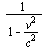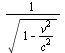## The Michelson-Morley Experiment

The Michelson-Morley experiment involved no measurement of the one way speed of light. It cannot therefore tell us anything about the one way speed of light relative to the experiment's frame of reference. What it does accomplish is a test for independence, with respect to orientation, of length determined by interferometry of a given solid object. Actually, the orientations of two perpendicular lengths, approximately equal, were interchanged without discernibly altering the difference of their interferometric lengths.

Calculations of a predicted dependence were based on some assumptions: (1) There is a unique frame of reference (the luminiferous ether or rest frame) relative to which light speed is c in every direction. (2) The experimental apparatus is moving at considerable speed relative to the ether. (3) Length dimensions of a solid are constant and unaffected by motion relative to the ether. According to these assumptions the rest frame distance the interferometer light travels is longer for an interferometer in motion, relative to one at rest, by the factorparallel to the motion and by the factorperpendicular thereto. It is now usual to recognize the falsity of the third assumption. The theory being here developed maintains the first and second assumptions, and modifies only the third.

Einstein's Special Relativity changes all three assumptions. The first Einstein made false by definition. The second he thus robbed of meaning. The third he modified to assert that length dimensions perpendicular to motion of a solid are constant and unaffected by the motion. Yet, Einstein's modified assumptions themselves, though consistent with the Michelson-Morley null result, do not satisfactorily explain it. His "simultaneous events" are not well defined, and there are no observations of simultaneous events in the experiment. His length contraction is without physical explanation, and there is no relative motion of apparatus and observer in the experiment.

The outcome of the Michelson-Morley experiment was that interferometric length is equivalent to measuring stick length independent of orientation. This must be true for the SI standard of length to work, because interferometry is used to calibrate gage blocks.

A simple, elegant and highly plausible conjecture explains the Michelson-Morley null result. Suppose that the forces that govern the dimensions of a solid are the consequence of an interferometer-like process in the sense that the equilibrium molecular bond lengths and crystal cell dimensions are such as will be spanned by a constant round trip electromagmetic wave count of constant frequency, or equivalently by a round trip of light in a fixed characteristic time interval. This is our new third assumption. Accordingly, a solid rod contracts by the factorparallel to the absolute velocity v, and byperpendicular thereto.

It should be noted that these factors differ from the corresponding factors,and 1, respectively, proposed ad hoc by Lorentz. (FitzGerald, by contrast, suggested contraction both parallel and perpendicular by a direction dependent amount.) Both sets of factors appear in Einstein's “Zur Elektrodynamik bewegter Körper” (1905) with the spurious claim that “substituting for x' what it equals” achieves the transition from the one to the other. If the Lorentz contraction factors were correct, the U. S. A. National Bureau of Standards interferometric method of determining length would, in principle, find that the length of a gage block, though stable with respect to orientation, would be unstable with changes of velocity, (time of day, time of year,) unless for increasing velocity there is a compensating decrease of laser frequency (time dilation). With the conjectured transformations, gage block length would be stable without time dilation. It is interesting that Einstein derived time dilation free transformations first, before inexplicably switching to Lorentz transformations.

 Michelson, Albert Abraham & Morley, Edward Williams (1887). “On the Relative Motion of the Earth and the Luminiferous Ether.” American Journal of Science 34: 333-345. online http://en.wikisource.org/wiki/On_the_Relative_Motion_of_the_Earth_and_the_Luminiferous_Ether.

 Doiron, Ted and Beers, John, The Gauge Block Handbook, NIST (1995), online http://www.nist.gov/calibrations/upload/mono180.pdf.

TOP: Physics Fixes Home

UP: Appendix--An Algorithm for Determination of Absolute Velocity

PREVIOUS: De Sitter's Astronomical Proof of the Constancy of Light Speed

NEXT: Definitions and Notation